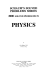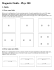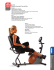# Pirate Vision. - Little Shop of Physics

## Transcription

Pirate Vision. - Little Shop of Physics
```Pirate Vision
Power of
visual
system
Power (D)
60
61
62
63
64
65
70
1
1
= 60 +
f
s
Distance (m)
∞
1.0
0.50
0.33
0.25
0.20
0.10
Viewing
distance
A myopic man
has a far point
of 50 cm.
What power
lens does he
need?
With my -5.0 D corrective lenses, my distant
vision is quite sharp; my near point is 1.0 m.
I have a pair of -3.5 D computer glasses that put
my computer screen right at my near point.
• How far away is my computer?
• What is my far point with these glasses on?
Reviewing.
Electricity, Magnetism: 5 Key Concepts
• Charge & charges in matter
• The field model
‣Electric field exerts force on charges, magnetic field exerts force on
moving charges.
‣Electric field due to charge distribution, magnetic field due to
currents.
• Potential & circuits
KNIG5491_02_ch26_pp852-883.qxd
8/17/09
3:10 PM
Page 880
‣Basic circuit, resistance
‣More complex circuits
PART
VI
SUMMARY
• Changing magnetic field induces emf
Electricity
wavesand Magnetism
• Electromagnetic
Mass and charge are the two most fundamental properties
‣Nature
of matter. of
The waves
first four parts of this text were about
properties and interactions of masses. Part VI has been a
of waves
‣Spectrum
study of charge—what
charge is and how charges interact.
Electric and magnetic fields are real and can exist
independently of charges. The clearest evidence for their
independent existence is electromagnetic waves—the
quintessential electromagnetic phenomenon. All electromagnetic waves, including light, are similar in structure, but
they span a wide range of wavelengths and frequencies. In
Part VI, we got our first hints that electromagnetic waves
might have a particle-like nature, a concept we will explore
further in Part VII.
Part VI has introduced many new phenomena, concepts,
and laws. The knowledge structure below draws together the
major ideas. These ideas build on what we’ve learned in the
first five parts of this text. All the pieces are now in place to
support the development of the ideas of modern physics in
Part VII.
Electric and magnetic fields were introduced to enable us
to understand the long-range forces of electricity and
magnetism. One charge—the source charge—alters the
space around it by creating an electric field and, if the charge
is moving, a magnetic field. Other charges experience forces
exerted by the fields. Electric and magnetic fields are the
agents by which charges interact.
In addition to the electric field, we often describe electric
interactions in terms of the electric potential. This is a
particularly fruitful concept for dealing with electric circuits
in which charges flow through wires, resistors, etc.
KNOWLEDGE STRUCTURE VI
Electricity and Magnetism
BASIC GOALS
How do charged particles interact? How do electric circuits work?
What are the properties and characteristics of electric and magnetic fields?
GENERAL
PRINCIPLES
Forces between charges:
Coulomb’s law
F1 on 2 = F2 on 1 =
Electric force on a
charge:
F = qE
The force is in the direction of the field for a positive
charge; opposite the field for a negative charge.
Magnetic force on a
moving charge:
F = ƒ q ƒ vB sin a
The force is perpendicular to the velocity and the
field, with direction as specified by the right-hand rule
for forces.
Induced emf:
B
E= `
K ƒ q1 ƒ ƒ q2 ƒ
r2
B
The force is along the line connecting the charges. For
like charges, the force is repulsive; for opposite
charges, attractive.
The induced current I = E/R is such that the induced
magnetic field opposes the change in the magnetic
flux. This is Lenz’s law.
¢£
`
¢t
Electric and magnetic fields
Current and circuits
Charges and changing magnetic fields create electric fields.
Potential differences ¢V drive current in circuits. Though
electrons are the charge carriers in metals, the current I is
defined to be the motion of positive charges.
• Electric fields exert forces on charges and torques on dipoles.
• The electric field is perpendicular to equipotential surfaces and
points in the direction of decreasing potential.
• The electric field causes charges to move in conductors but not
insulators.
Currents and permanent magnets create magnetic fields.
• Magnetic fields exert forces on currents (and moving charged
particles) and torques on magnetic dipoles.
• A compass needle or other magnetic dipole will line up with a
magnetic field.
Battery
Resistor
I
1
DVbat
r
E
2
• Circuits obey Kirchhoff’s loop
law (conservation of energy)
and Kirchhoff’s junction law
(conservation of charge).
• The current through a resistor
is I = ¢VR /R. This is Ohm’s
law.
Electromagnetic waves
Electric potential
The interaction of charged particles can also be described in
terms of an electric potential V.
• Only potential differences ¢V are important.
• If the potential of a particle of charge q changes by ¢V, its
potential energy changes by ¢U = q¢V.
• Where two equipotential surfaces with potential difference ¢V
are separated by distance d, the electric field strength is E = ¢V/d.
880
An electromagnetic wave is a self-sustaining oscillation of
electric and magnetic fields.
B
B
• E and B are perpendicular to each other and to the direction
of travel.
• All electromagnetic waves travel at the same speed, c.
• The electromagnetic spectrum is the spread of
wavelengths and frequencies of electromagnetic waves,
from radio waves through visible light to gamma rays.
A proton is released from
rest at the dot. Afterward,
the proton
Remains at the dot.
speed.
C. Moves upward with an increasing speed.
D. Moves downward with a steady speed.
E. Moves downward with an increasing speed.
A.
B.
A metal bar moves through a
magnetic field. The induced charges
on the bar are
Modern Physics: 3 Key Concepts
• Quantum notions
‣Particle nature of light
‣Wave nature of matter
• Quantum consequences
‣Discrete energy levels & transitions
• Nuclear physics:
‣Decay
know an electron’s position and velocity at the same time.
Many decades of clever experiments have shown conclusively
that no underlying laws can restore the predictability of
Part VII summarizes the key ideas of relativity, quantum
physics, and nuclear physics. These are the theories behind
the emerging technologies of the 21st century.
Modern Physics
KNOWLEDGE STRUCTURE V
BASIC GOALS
What are the properties and characteristics of space and time?
What do we know about the nature of light and atoms?
How are atomic and nuclear phenomena explained by energy levels, wave functions, and photons?
GENERAL PRINCIPLES
Principle of relativity
Quantization of energy
Uncertainty principle
Pauli exclusion principle
All the laws of physics are the same in all inertial reference frames.
Particles of matter and photons of light have only certain allowed energies.
¢ x ¢p Ú h/2p
No more than one electron can occupy the same quantum state.
Relativity
• The speed of light c is the same in all inertial reference frames.
• No particle or causal influence can travel faster than c.
• Length contraction: The length of an object in a reference
frame in which the object moves with speed v is
L = 21 - b 2 / … /
where / is the proper length.
• Time dilation: The proper time interval ¢t between two
events is measured in a reference frame in which the two
events occur at the same position. The time interval ¢t in a
frame moving with relative speed v is
¢t = ¢t/ 21 - b 2 Ú ¢t
• Particles have energy even when at rest. Mass can be
transformed into energy and vice versa: E 0 = mc2.
Quantum physics
• Matter has wave-like properties. A particle has a de Broglie
wavelength:
h
l=
mv
• Light has particle-like properties. A photon of light of
frequency f has energy:
hc
Ephoton = hf =
l
• The wave nature of matter
levels in atoms and nuclei.
A transition between quantized
energy levels involves the
emission or absorption of a photon.
Properties of atoms
• Quantized energy levels depend on quantum numbers n and l.
• An atom can jump from one state to another by emitting or absorbing a photon of energy Ephoton = ¢Eatom.
• The ground-state electron configuration is the lowest-energy configuration consistent with the Pauli principle.
Properties of nuclei
• The nucleus is the small, dense,
positive core at the center of an
atom. The nucleus is held together
by the strong force, an attractive
short-range force between
any two nucleons.
Proton
Neutron
• Unstable nuclei decay by
alpha, beta, or gamma
decay. The number of
nuclei decreases
exponentially with time:
1 t/t1/2
N = N0 a b
2
1024
N
N0
0.50N0
0.37N0
0
0
t1/2
t
t
4th PROOF
An atom has the energy levels shown. Do you expect to see a
spectral line with wavelength of 620 nm in this atom’s emission
spectrum?
A. Yes.
B. No.
C. There’s not enough
information to tell.
Optics: 3 Key Concepts
• Wave properties:
‣Interference
‣Diffraction
• Reflection & refraction
• Optics:
‣Lenses & images
‣Vision and vision correction
wavelengths of visible light are so short. Wave phenomena
become apparent only when light interacts with objects or
holes whose size is less than about 0.1 mm.
KNOWLEDGE STRUCTURE V
formation with lenses and mirrors. We found that the
ultimate resolution of an optical instrument is set by the
wave nature of light, bringing our study of optics full circle.
Optics
BASIC GOALS
What are the consequences of the wave nature of light?
In the ray model, how do light rays refract and reflect to form images?
GENERAL PRINCIPLES
Light is understood using two models, the wave model, in which light exhibits wave properties such as interference and diffraction, and the ray model, in which light travels in straight lines until it reflects or refracts.
Wave model
Ray model
• Light travels out from its source in straight lines, called rays.
• Light spreads out when passing through
a narrow opening. This is diffraction.
Screen
The intensity on a screen consists
of a bright central maximum and
fainter secondary maxima.
a
w
The width of the central
maximum is
2lL
w 5 ___
a
L
• Light waves from multiple slits in a screen interfere where
they overlap. The light intensity is large where the interfering
waves are in phase, and small where they are out of phase.
The intensity on a screen
consists of equally spaced
interference fringes.
Dy
d
The fringe
spacing is
• Light rays change direction as
they cross the surface between
two media. The angles of
incidence and refraction are
related by Snell’s law:
ui ur
Incident
u1
ray
n1
n2
u2
n1 sin u1 = n2 sin u2
where n is the index of refraction. The speed of light in a
transparent material is v = c/n.
Image formation by lenses and mirrors
• Light waves reflected from the two surfaces of a thin transparent film also interfere.
The resolution of optical instruments
For a microscope, the miniDiffraction limits how
close together two
mum resolvable distance
point objects can be
between two objects is
and still be resolved.
For a telescope, the minimum
resolvable angular separation
between two objects is
1.22l
u1 =
D
0.61l
NA
where the numerical aperture
NA is a characteristic of the
microscope objective.
dmin =
Image
Object
f
s
A lens creates an
image as shown. In
this situation,
B.
C.
D.
s < f.
f < s < 2f.
s > 2f.
There’s not enough information
to compare s to f.
The Eye
f
s9
The object distance s, the image distance s¿, and the focal
length are related by the thin-lens equation, which also works
for mirrors:
4th PROOF
A.
Refracted
ray
A lens or mirror has a characteristic focal length f. Rays parallel to
the optical axis come to focus a distance f from the lens or mirror.
lL
Dy 5 __
d
L
• Rays reflect off a surface
between two media, obeying
the law of reflection, ui = ur .
1 1 1
+ =
s s¿ f
Underwater vision correction?
Air lens in water.
Converging or diverging?
Horse Sense
The ciliary muscles in a horse’s eye can only make small changes to the shape
of the lens, so a horse can’t change the shape of the lens to focus on objects at
different distances as humans do. Instead, a horse relies on the fact that its
eyes aren’t spherical. As the figure shows, different points at the back of the
eye are at somewhat different distances from the front of the eye.
We say that the eye has a
“ramped retina”; images that
form on the top of the retina are
at a greater distance from the
cornea and lens than those that
form at lower positions. The
horse uses this ramped retina to
focus on objects at different
distances, tipping its head so that
light from an object forms an
image at a vertical location on
the retina that is at the correct
distance for sharp focus.
Lens
Iris
Cornea
m
45 m
43 mm
Retina
40 m
m
In a horse’s eye, the image of a close object will be in focus
! A.!! At the top of the retina.
! B.!! At the bottom of the retina.
In a horse’s eye, the image of a distant object will be in focus
! A.!! At the top of the retina.
! B.!! At the bottom of the retina.
Lens
Iris
Cornea
m
45 m
43 mm
40 m
m
Retina
A horse is looking straight ahead at a person who is standing quite
close. The image of the person spans much of the vertical extent of
the retina. What can we say about the image on the retina?
!
!
!
!
A.! The person’s head is in focus; the feet are out of focus.
B.! The person’s feet are in focus; the head is out of focus.
C.!The person’s head and feet are both in focus.
D.! The person’s head and feet are both out of focus.
Lens
Iris
Cornea
m
45 m
43 mm
Retina
40 m
m
Certain medical conditions can change the shape of a horse’s eyeball;
these changes can affect vision. If the lens and cornea are not changed
but all of the distances in the figure are increased slightly, this will
make the horse
!
!
!
A.! Nearsighted.
B.! Farsighted.
C.!Unable to focus clearly at any distance.
Lens
Iris
Cornea
m
45 m
43 mm
Retina
40 m
m
rhodopsin in rod cells
(responsible for vision in
dim light) has a threshold
photon energy of 1.8 eV.
Explain why astronomers
use red flashlights.
```

### online_test_u11_test..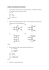### Key HW5_2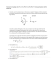### Medizinische Austellung A4 Wien 2008.indd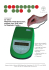### Pig Eye Dissection - St. - Ursula - Gymnasium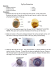### Problem Set 08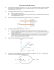### SAMPLE PAPER – I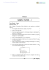### Ph 632 Problem Set #4 - Department of Physics | Oregon State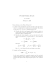### 3000 solved problems in physics